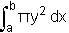##### Basic Math & Pre-Algebra For Dummies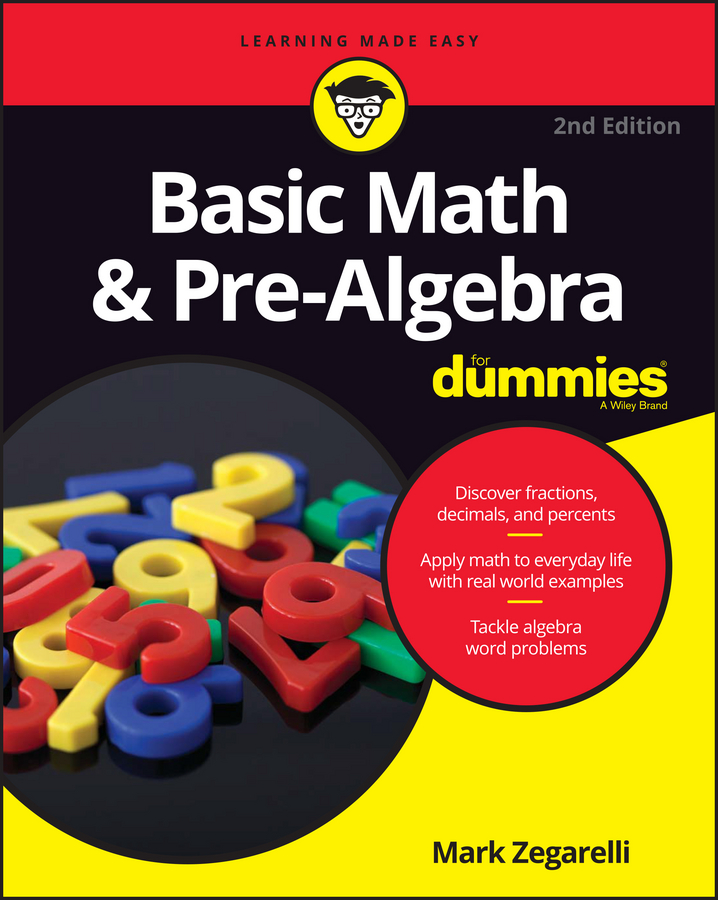Some of the most important things to remember in AS-level and A-level maths are the rules for differentiating and integrating expressions. This cheat sheet is a handy reference for what happens when you differentiate or integrate powers of x, trigonometric functions, exponentials or logarithms – as well as the rules you need for what to do when they’re combined!

## Differentiating simple terms and functions

This is a handy guide to the building blocks of differentiation for A-level maths: it gives you all of the things you’re expected to know to differentiate (powers of x, exponentials, logarithms and trigonometric functions) and what you get when you do the calculus!

In the following table, a represents any constant.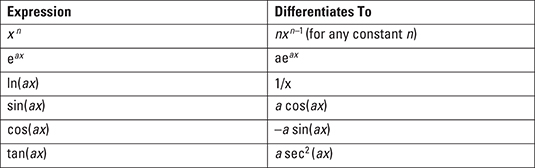Note that ln(ax) differentiates to a / ax, and after you cancel the as you’re left with 1/x.

## Differentiating combinations

Once you’ve mastered the building blocks of differentiation for A-level maths, the next step is to get comfortable with the various rules – what happens when you have a function with something complicated inside the brackets? How about if you have expressions involving addition, subtraction, multiplication or division? This table reminds you what to do in these situations, including the dreaded chain rule, quotient rule and product rule from Core 3.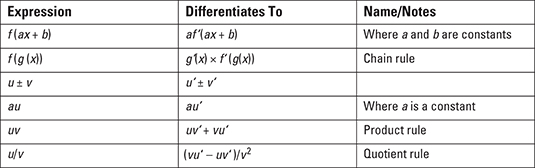If you’re doing parametric differentiation in Core 4, then dy/dx = (dy/dt)/(dx/dt).

## Integrating simple terms and functions

If you want to get a good grade in A-level maths, one of the skills you need is the integration of simple terms – powers of x, exponentials and trigonometric functions.

Any time you integrate, you have to remember to add a constant! If you are working with limits, this cancels out, and if you have additional information, you may be able to work the constant out.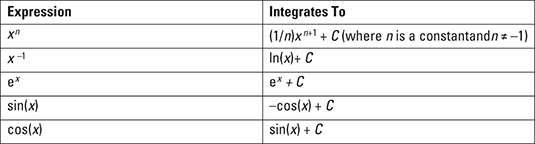## Integrating combinations

When you have to integrate something more complicated than a simple expression for A-level maths, the chances are you’ll need to use one of the rules in this table, which shows you how to deal with a function when it has a linear argument, two terms added to or subtracted from each other, and the formula for integration by parts. In this table, a capital letter means “the integral of the lower-case letter” – so F is the integral of f, U is the integral of u, and V is the integral of v.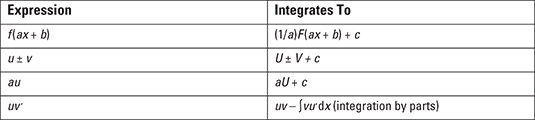You also need to know about calculating a volume of revolution: Rotating the curve y=f(x) around the x-axis between limits of a and b gives you a volume of